ML Aggarwal Class 6 Solutions Chapter 14 Mensuration Ex 14.1 for ICSE Understanding Mathematics acts as the best resource during your learning and helps you score well in your exams.

## ML Aggarwal Class 6 Solutions for ICSE Maths Chapter 14 Mensuration Ex 14.1

Question 1.
Find the perimeter of each of the following figures: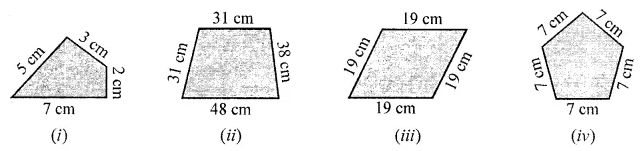Solution:Question 2.
Find the perimeter of each of the following shapes:
(i) A triangle of sides 3 cm, 4 cm and 6 cm.
(ii) A triangle of sides 7 cm, 5.4 cm and 10.2 cm.
(iii) An equilateral triangle of side 11 cm.
(iv) An isosceles triangle with equal sides 10 cm each and third side 7 cm.
Solution: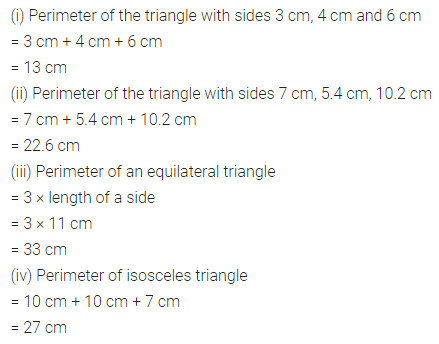Question 3.
The lid of a rectangular box of sides 40 cm by 10 cm is sealed all round with tape. What is the length of the tape required?
Solution:Question 4.
Table-Top measures 2 m 25 cm by 1 m 50 cm. What is the perimeter of the table-top?
Solution: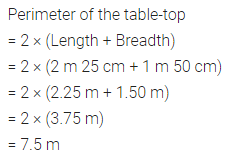Question 5.
A rectangular piece of land measures 0.7 km by 0.5 km. Each side is to be fenced with 4 rows of wires. What is the length of the wire needed?
Solution: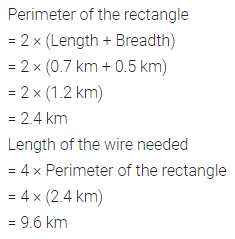Question 6.
Find the perimeter of a regular hexagon with each side measuring 7.5 m.
Solution:Question 7.
The lengths of two sides of a triangle are 12 cm and 14 cm. The perimeter of the triangle is 36 cm. What is the length of its third side?
Solution: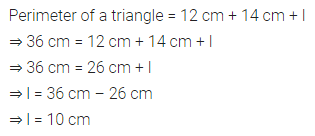Question 8.
The perimeter of a regular pentagon is 100 cm. How long is its every side?
Solution:Question 9.
A piece of string is 30 cm long. What will be the length of each side if the string is used to form:
(a) a square?
(b) an equilateral triangle?
(c) a regular hexagon?
Solution: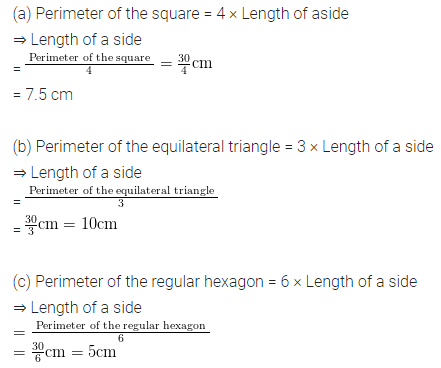Question 10.
Find the cost of fencing a rectangular park of length 225 m and breadth 115 m at the rate of ₹13 per metre.
Solution: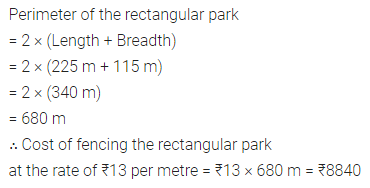Question 11.
Meera went to a rectangular park 140 m long and 90 m wide. She took 5 complete rounds on its boundary. What is the distance covered by her?
Solution: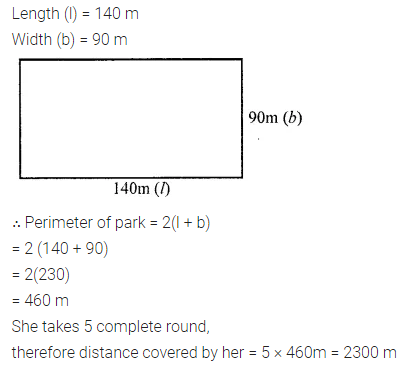Question 12.
Pinky runs 8 times around a rectangular park with length 80 m and breadth 55 m while Pankaj runs 7 times around a square park of side 75 cm. Who covers more distance and by how much?
Solution: